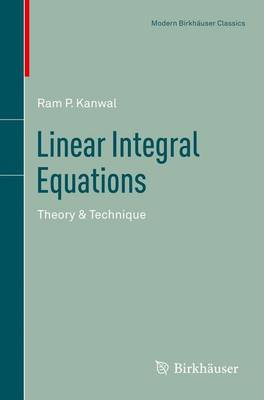•# Linear Integral Equations: Theory & Technique - Modern Birkhauser Classics (Paperback)

(author)
£54.99
Paperback 318 Pages / Published: 07/11/2012
• We can order this from the publisher

Usually dispatched within 15 working days

Many physical problems that are usually solved by differential equation methods can be solved more effectively by integral equation methods. Such problems abound in applied mathematics, theoretical mechanics, and mathematical physics. This uncorrected soft cover reprint of the second edition places the emphasis on applications and presents a variety of techniques with extensive examples.Originally published in 1971, Linear Integral Equations is ideal as a text for a beginning graduate level course. Its treatment of boundary value problems also makes the book useful to researchers in many applied fields.

Publisher: Birkhauser Boston Inc
ISBN: 9781461460114
Number of pages: 318
Weight: 5037 g
Dimensions: 235 x 155 x 17 mm
Edition: 2nd ed. 2013

MEDIA REVIEWS

A nice introductory text... Presents the basics of linear integral equations theory in a very comprehensive way... [The] richness of examples and applications makes the book extremely useful for teachers and also researchers.

-Applications of Mathematics (Review of the Second Edition)

This second edition of this highly useful book continues the emphasis on applications and presents a variety of techniques with extensive examples...The book is ideal as a text for a beginning graduate course. Its excellent treatment of boundary value problems and an up-to-date bibliography make the book equally useful for researchers in many applied fields.

-MathSciNet (Review of the Second Edition)